成像光学 物理光学 光纤激光 照明 杂散光 二次开发 课程资讯 教学实验室 书籍中心 技术文章 常见问题 联系我们 工程顾问 光学成像设计 SYNOPSYS光学设计软件 ASAP光学设计软件 RP Fiber Power 平场聚焦透镜F-THETA SCAN LENS Mathematica全球现代技术计算终极系统 光学加工 PCGrate 光栅设计软件 APEX 光学分析设计软件#### MATHEMATICA 全球现代技术计算的终极系统

Mathematica 在其三十年的开发历程中，在技术计算领域确立了最先进的技术，并为全球技术创新人员、教育工作者、学生和其他人士提供了最主要的计算环境。

Mathematica 以卓越的技术和简便的使用方法享誉全球，在此基础上，它提供了单个集成并且持续扩展的系统，涵盖了最广最深的技术计算功能，并可通过网页浏览器实现云端的完美访问，以及在所有现代桌面系统上的本地访问。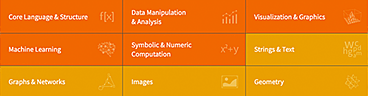Mathematica 具有涵盖所有技术计算领域的将近 6,000 个内置函数——所有这些都经过精心制作，使其完美地整合在 Mathematica 系统中。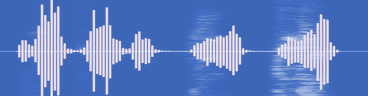Mathematica 在所有领域构建了前所未有的强大算法—许多算法都是使用 Wolfram 语言独特的开发方法和功能进行构建的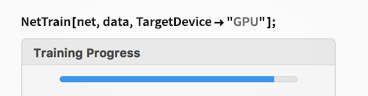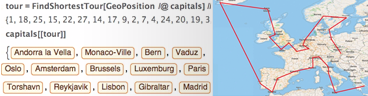Mathematica 凭借它的算法功能以及 Wolfram 语言的详细设计原理，创建了具有预测性建议、自然语言输入等的独特的并且易于使用的系统。

Mathematica 使用 Wolfram 笔记本界面，使您可以快速整理包括文本、可运行代码、动态图形和用户界面等的丰富文档中的任何内容。

Mathematica 使用最先进的计算美学和设计原理，为你呈现最美观的结果；立即创建最顶级的互动可视化效果和出版物质量级别的文档。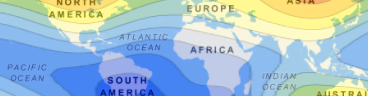Mathematica 可以访问广博的 Wolfram 知识库，包括最实时的数千个领域的数据。Mathematica 目前已经完美地集成于云端系统中；可在统一强大的云端桌面混合环境中进行分享、云计算以及更多功能。Mathematica 为与任意内容连接而构建：文件格式（180 多种）、其他语言、 Wolfram Data Drop、API、数据库、程序、物联网和设备，甚至其自身分布等。

 Mathematica 基于具有突破性意义的 Wolfram 语言。 可视化 代数操作 数论 数据分析 图形计算 交互式计算 图像计算 几何计算 导入和导出

WOLFRAM 语言

WOLFRAM 算法库

WOLFRAM 笔记本界面

WOLFRAM ENGINE

WOLFRAM CLOUD

WOLFRAM KNOWLEDGEBASE

Mathematica 发展轨迹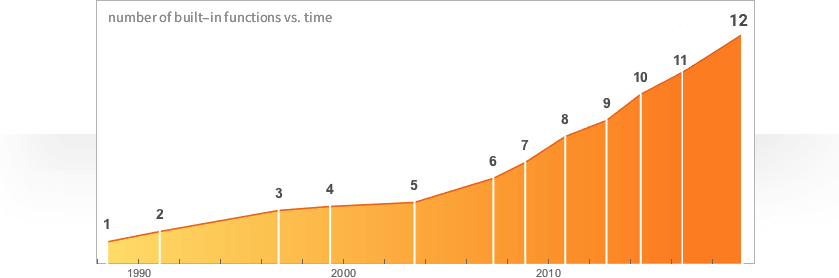1988 年革新

Mathematica 在三十年多的时间里遵从快速革新的发展轨迹，使得在每个阶段都构建了许多强大的新功能。

Mathematica 的各版本更新不仅仅是一般的软件更新；每个连续更新的版本都是在新方向上对计算模式的一次重大发展，并且引入了重要的新思路。

Mathematica 忠实于它的核心准则和严肃的设计原理，持续发展并且集成了许多新功能和方法，而无需走回头路。

Mathematica 是 Wolfram 独创的旗帜产品；旨在为研发和教育工作提供技术计算平台。基于 Wolfram 语言，Mathematica 与其他核心 Wolfram 产品可以百分百兼容。

Mathematica 应用可应用于有限元环境分析寻找高级全局和局部非线性最优化生成多语言、多环境数值代码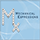设计符号式力学系统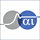模拟电子电路的智能符号式设计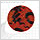运动学和动力学分析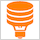数据库编辑训练和分析神经网络，便于拟合数据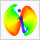简化数据分析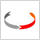面向对象的仿真拟合、可视化和误差分析处理用于求解广泛范围的最优化问题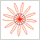用于精确绘图和几何学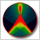设计和分析自定义光学系统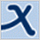互动符号式几何模拟和数字系统的原理图捕获、符号式求解、处理和实现约束和非约束非线性函数的全局优化<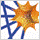桌面超级计算光线追迹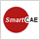热传导仿真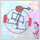创建机械结构原型系统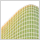面向应用程序的统计推断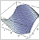创建最优化 C++ 代码运行具有上百或者上千分量的张量计算生成最优化 Fortran 90 程序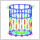框架结构的互动三维结构化分析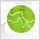将 Mathematica 的强大功能带入 Excel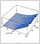快速和精确分析数学高级建模和优化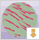微分方程可视化